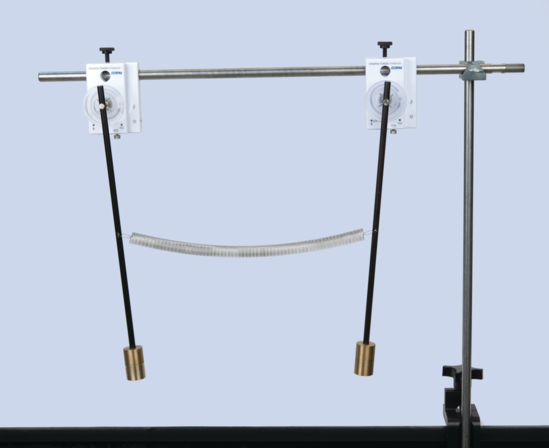# Coupled Pendulum (PASCO)

Coupled Pendulum EX-5563

Coupled pendula exchange oscillation energy.

Two pendula are coupled by a spring. This system has two natural modes:

• The two pendula swing in phase with each other.

• The two pendula swing 180 degrees out of phase.

When one of the pendula is held at rest and the other is set oscillating, the energy of the oscillating pendulum is transferred to the other pendulum by the spring. The period of the energy transfer can be predicted and verified by experiment.In this experiment, the motion of each pendulum is tracked using a Wireless Rotary Motion Sensor. Since the angles are small, the equations of motion yield the following equation for the period:5 次查看0 則留言